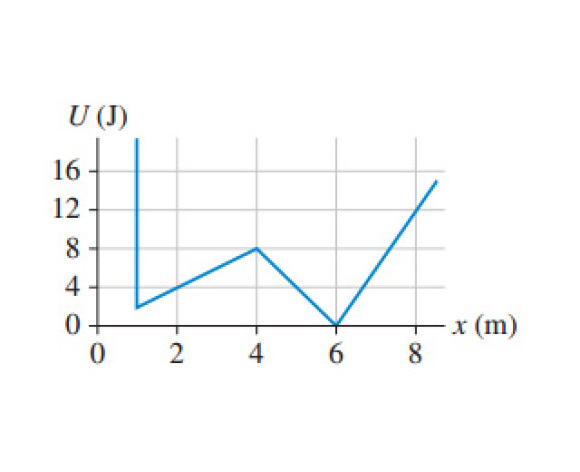# [SOLVED] Mastering Physics Help PLEASE!!!?

In (Figure 1) a particle has a mass of 280 g. What is the maximum speed the particle could have at x = 2.0 m and never reach x = 6.0 m?

Express your answer to two significant figures and include the appropriate units.by
selected by

4u just a guess aha

Lorem ipsum dolor sit amet, consectetur adipiscing elit, sed do eiusmod tempor incididunt ut labore et dolore magna aliqua. Ut enim ad minim veniam, quis nostrud exercitation ullamco laboris nisi ut aliquip ex ea commodo consequat. Duis aute irure dolor in reprehenderit in voluptate velit esse cillum dolore eu fugiat.
by

The particle has 4J of potential energy at x = 2m (read from the graph). As the particle moves from x = 2 m to x = 4 m , it is losing kinetic energy and gaining potential energy. Since the total mechanical energy is constant, (mechanical energy = sum of kinetic + potential energy), the particle would need to have 8J of energy to reach x = 4 m. This would occur when the potential energy = 8 J and kinetic energy = 0 J. If the particle has more potential energy than 8J, it could easily make it to x = 6 m. Therefore, our maximum mechanical energy is 8 J.

Returning to x = 2 m, if the mechanical energy = 8 J and the potential energy = 4 J, that must mean that the kinetic energy is equal to 4 J. The velocity can be calculated by setting 1/2mv^2 = 8 J, and substituting m = 0.280 kg, giving v = 5.35 m/s.

Lorem ipsum dolor sit amet, consectetur adipiscing elit, sed do eiusmod tempor incididunt ut labore et dolore magna aliqua. Ut enim ad minim veniam, quis nostrud exercitation ullamco laboris nisi ut aliquip ex ea commodo consequat. Duis aute irure dolor in reprehenderit in voluptate velit esse cillum dolore eu fugiat.

+1 vote